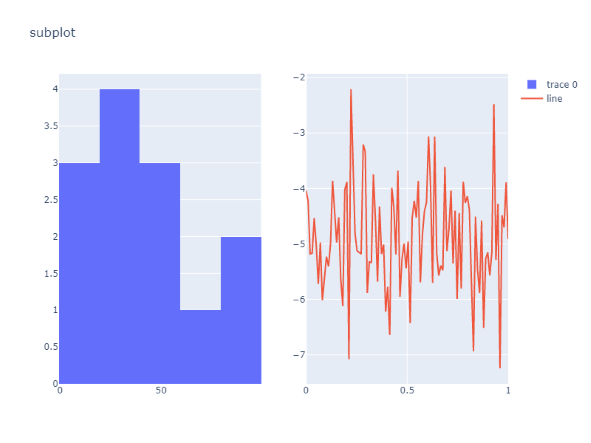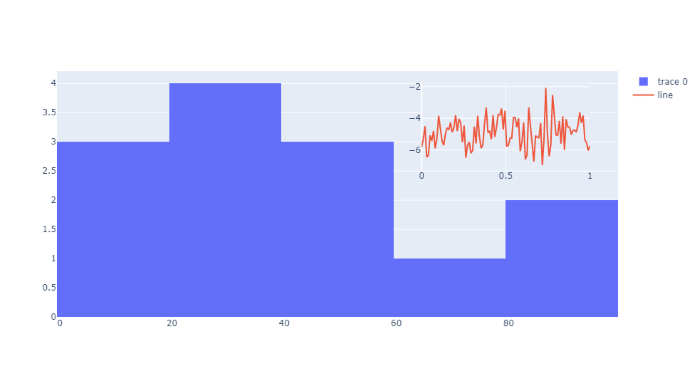# Python Plotly – Subplots and Inset Plots

Perquisites: Python Plotly

One of the most deceptively-powerful features of Plotly data visualization is the ability for a viewer to quickly analyze a sufficient amount of information about data when pointing the cursor over the point label appears.

Syntax: layout = go.Layout( xaxis2 = dict( domain = [0-1], anchor = ‘axis’)

Where:

• xaxis2: list
• anchor: position_axis

### Example 1: Plotly Subplots

The subplots method provides a way to plot multiple plots on a single figure. This supports the concept of subplots which offer function in plotly.tools module. The function returns a Figure object. In this example, we are taking two data plots, the first one is a Line chart and another is a Histogram chart.

## Python3

 `from` `plotly ``import` `tools` `import` `plotly.graph_objs as go` `from` `plotly.offline ``import` `iplot` `import` `numpy as np`   `# defining data for chart 1` `x1 ``=` `np.array([``22``, ``87``, ``5``, ``43``, ``56``, ``73``, ``11``,` `               ``42``, ``20``, ``5``, ``31``, ``27``, ``85``])`   `# defining data for chart 2` `N ``=` `100` `x_vals ``=` `np.linspace(``0``, ``1``, N)` `y_vals ``=` `np.random.randn(N) ``-` `5`   `# chart 1` `chart1 ``=` `go.Histogram(x``=``x1)`   `# chart 2` `chart2 ``=` `go.Scatter(` `    ``x``=``x_vals,` `    ``y``=``y_vals,` `    ``mode``=``'lines'``,` `    ``name``=``'line'``,` `    ``xaxis``=``'x2'``,` `    ``yaxis``=``'y2'``,` `)` `fig ``=` `tools.make_subplots(rows``=``1``, cols``=``2``)` `fig.append_trace(chart1, ``1``, ``1``)` `fig.append_trace(chart2, ``1``, ``2``)` `fig[``'layout'``].update(height``=``600``, width``=``800``,` `                     ``title``=``'subplot'``)` `iplot(fig)`

Output:### Example 2: Plotly Inset Plots

An Inset graph is a pictorial representation of values and data. It is a chart that is added to an existing chart in the given subplot graph. The dimensions of the inset chart are reduced so that the host plot remains at least partially visible in the graph plot. It provides us with the easiest way to read and analyze information from the document.

In this example, we are taking two data plots, first one is a Line chart and another is a Histogram chart which is assigned as chart1 and chart2 are passed as a list in the layout method. The x-axis and y-axis properties of inset trace to ‘x2’ and ‘y2’ respectively.

Now, an x-axis can be anchored to a y-axis, for example, xaxis2: {anchor: ‘y2’} means that xaxis2 joins yaxis2 at the origin. If anchors are set to free, the position attribute can then be used to move the axis to another position.

## Python3

 `from` `plotly ``import` `tools` `import` `plotly.graph_objs as go` `from` `plotly.offline ``import` `iplot` `import` `numpy as np`   `# defining data for chart 1` `x1 ``=` `np.array([``22``, ``87``, ``5``, ``43``, ``56``, ``73``, ``11``,` `               ``42``, ``20``, ``5``, ``31``, ``27``, ``85``])`   `# defining data for chart 2` `N ``=` `100` `x_vals ``=` `np.linspace(``0``, ``1``, N)` `y_vals ``=` `np.random.randn(N) ``-` `5`   `# chart 1` `chart1 ``=` `go.Histogram(x``=``x1)`   `# chart 2` `chart2 ``=` `go.Scatter(` `    ``x``=``x_vals,` `    ``y``=``y_vals,` `    ``mode``=``'lines'``,` `    ``name``=``'line'``,` `    ``xaxis``=``'x2'``,` `    ``yaxis``=``'y2'``,` `)`   `data ``=` `[chart1, chart2]`   `# setting layout` `layout ``=` `go.Layout(`   `    ``# setting y-axis position for chart 2` `    ``xaxis2``=``dict``(` `        ``domain``=``[``0.65``, ``0.95``],` `        ``anchor``=``'y2'` `    ``),`   `    ``# setting y-axis position for chart 2` `    ``yaxis2``=``dict``(` `        ``domain``=``[``0.6``, ``0.95``],` `        ``anchor``=``'x2'` `    ``)` `)`   `fig ``=` `go.Figure(data``=``data, layout``=``layout)` `iplot(fig)`

Output:Whether you're preparing for your first job interview or aiming to upskill in this ever-evolving tech landscape, GeeksforGeeks Courses are your key to success. We provide top-quality content at affordable prices, all geared towards accelerating your growth in a time-bound manner. Join the millions we've already empowered, and we're here to do the same for you. Don't miss out - check it out now!